# TypeError: float object cannot be interpreted as an integer5 min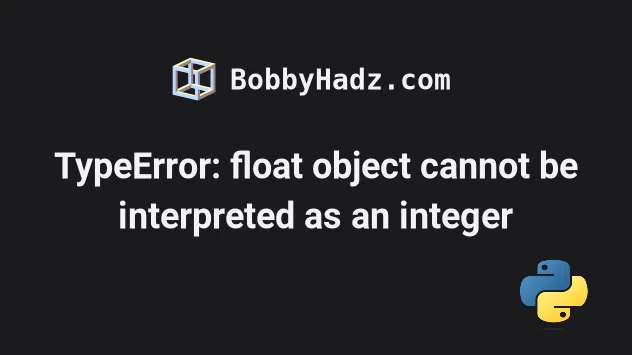## #TypeError: float object cannot be interpreted as an integer

The Python "TypeError: 'float' object cannot be interpreted as an integer" occurs when we pass a float to a function that expects an integer argument.

To solve the error, use the floor division operator, e.g. `for i in range(my_num // 5):`.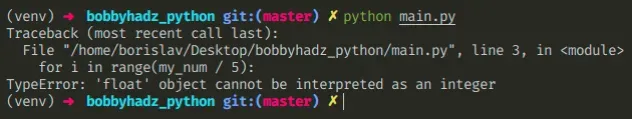Here is an example of how the error occurs.

main.py
```Copied!```my_num = 50

print(my_num / 5)  # 👉️ 10.0 (float)

# ⛔️ TypeError: 'float' object cannot be interpreted as an integer
for i in range(my_num / 5):
print(i)
``````

The division operator `/` always produces a float value but the `range()` function expects an integer.

Floating point numbers have one or more digits after the decimal, whereas integers don't have a decimal.

main.py
```Copied!```print(type(10.0))  # 👉️ <class 'float'>
print(type(10.5))  # 👉️ <class 'float'>

print(type(5))  # 👉️ <class 'int'>
print(type(13))  # 👉️ <class 'int'>
``````

## #Use the floor division operator to solve the error

To solve the error, use the floor division operator `//` instead of the division `/` operator.

main.py
```Copied!```my_num = 50

# ✅ using floor division
for i in range(my_num // 5):
print(i)

print(my_num / 5)  # 👉️ 10.0 (float)
print(my_num // 5)  # 👉️ 10 (int)

# ✅ or convert to int
print(int(10.0)) # 👉️ 10 (int)
``````

Division `/` of integers yields a float, while floor division `//` of integers results in an integer.

The result of using the floor division operator is that of a mathematical division with the `floor()` function applied to the result.

## #Convert the float to an integer when calling the function

Alternatively, you can convert the float to an int by passing it to the `int()` constructor.

main.py
```Copied!```my_num = 50

# ✅ convert float to int
for i in range(int(my_num / 5)):
print(i)

print(int(10.0))  # 👉️ 10
print(int(5.0))  # 👉️ 5
``````

The int class returns an integer object constructed from the provided number or string argument.

The constructor returns `0` if no arguments are given.

The range function is commonly used for looping a specific number of times in for loops and takes the following parameters:

NameDescription
`start`An integer representing the start of the range (defaults to `0`)
`stop`Go up to, but not including the provided integer
`step`Range will consist of every N numbers from `start` to `stop` (defaults to `1`)
main.py
```Copied!```print(list(range(5))) # 👉️ [0, 1, 2, 3, 4]
print(list(range(1, 5))) # 👉️ [1, 2, 3, 4]
``````

If you only pass a single argument to the `range()` constructor, it is considered to be the value for the `stop` parameter.

## #Convert the float to an integer by rounding

You can also convert the float to an integer by rounding.

main.py
```Copied!```my_float = 3.51

for i in range(round(my_float)):
print(i) # 0, 1, 2, 3

print(round(my_float)) # 👉️ 4
``````

The round function takes the following 2 parameters:

NameDescription
`number`the number to round to `ndigits` precision after the decimal
`ndigits`the number of digits after the decimal, the number should have after the operation (optional)

The `round` function returns the number rounded to `ndigits` precision after the decimal point.

If `ndigits` is omitted, the function returns the nearest integer.

You can use the `math.ceil()` or `math.floor()` method if you need to round the float up or down.

main.py
```Copied!```import math

my_float = 3.51

rounded_up = math.ceil(my_float)
print(rounded_up)  # 👉️ 4

rounded_down = math.floor(my_float)
print(rounded_down)  # 👉️ 3
``````

The math.ceil method returns the smallest integer greater than or equal to the provided number.

The math.floor method returns the largest integer less than or equal to the provided number.

If you are getting the error in a different scenario, you have to convert the float argument to an integer by passing it to the `int()` constructor.

## #Using `numpy.arange` to solve the error

The native `range()` function cannot be called with a floating-point number, but you can call the `numpy.arange` method with one.

main.py
```Copied!```import numpy as np

my_float = 3.5

for i in np.arange(my_float):
# 0.0
# 1.0
# 2.0
# 3.0
print(i)
``````

The numpy.arange method takes the `start`, `stop` and `step` values as parameters, just like the `range()` function.

However, `numpy.arange` handles floating-point values.

If you need to install numpy, open your terminal in your project's root directory and run the following command.

shell
```Copied!```pip install numpy

pip3 install numpy
``````

## #Reassigning a variable by mistake

Make sure you aren't declaring a variable that stores an integer initially and overriding it somewhere in your code.

main.py
```Copied!```my_int = 50

# 👇️ reassigned variable to a float by mistake
my_int = 5.6

# ⛔️ TypeError: 'float' object cannot be interpreted as an integer
for i in range(my_int):
print(i)
``````

We initially set the `my_int` variable to an integer but later reassigned it to a float which caused the error.

## #TypeError: 'str' object cannot be interpreted as an integer

The Python "TypeError: 'str' object cannot be interpreted as an integer" occurs when we pass a string to a function that expects an integer argument.

To solve the error, convert the string to an integer in the call to the function.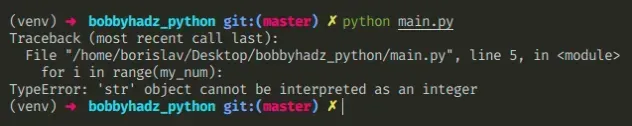Here is an example of how the error occurs.

main.py
```Copied!```# 👇️ this is a string
my_num = '5'

# ⛔️ TypeError: 'str' object cannot be interpreted as an integer
for i in range(my_num):
print(i)
``````

We passed a string to the `range()` constructor which expects an integer argument.

## #Convert the string to an integer in the call to the function

To solve the error, use the `int()` class to convert the string to an integer.

main.py
```Copied!```my_num = '5'

for i in range(int(my_num)):
print(i)
``````

The `int()` class returns an integer object constructed from the provided number or string argument.

main.py
```Copied!```print(int('5')) # 👉️ 5 (integer)
print(int('3')) # 👉️ 3 (integer)
``````

## #The input() function always returns a string

The error often occurs when taking input from the user with the built-in `input()` function.

main.py
```Copied!```# 👇️ this is a string
num = input('Enter your fav number: ')
print(num)
print(type(num))  # 👉️ <class 'str'>

# ⛔️ TypeError: 'str' object cannot be interpreted as an integer
for i in range(num):
print(i)
``````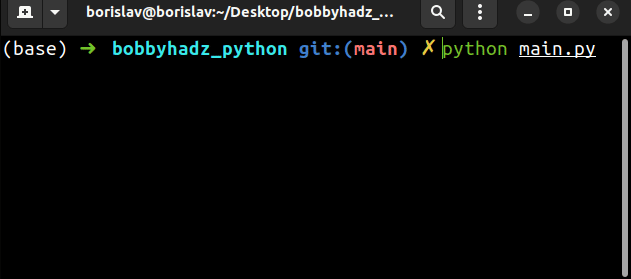The input function converts the supplied value to a string and returns it.

The `input()` function is guaranteed to return a string even if the user enters an integer.

Use the `int()` constructor to convert the value to an integer to solve the error.

main.py
```Copied!```num = input('Enter your fav number: ')
print(num)
print(type(num))  # 👉️ <class 'str'>

# ✅ Convert str to integer
for i in range(int(num)):
print(i)
``````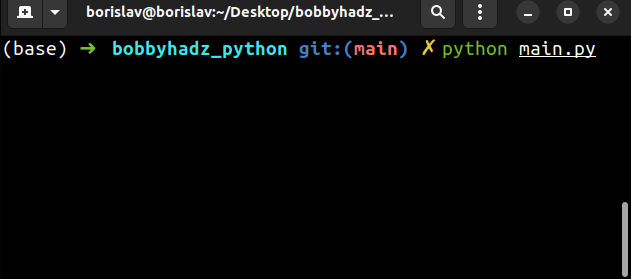The `int()` class returns an integer object constructed from the provided number or string argument.

The constructor returns `0` if no arguments are given.

main.py
```Copied!```print(int('10'))  # 👉️ 10
print(int('5'))  # 👉️ 5
``````

The range constructor is commonly used for looping a specific number of times in `for` loops and takes the following parameters:

NameDescription
`start`An integer representing the start of the range (defaults to `0`)
`stop`Go up to, but not including the provided integer
`step`Range will consist of every N numbers from `start` to `stop` (defaults to `1`)
main.py
```Copied!```print(list(range(3))) # 👉️ [0, 1, 2]
print(list(range(1, 4))) # 👉️ [1, 2, 3]
``````

If you only pass a single argument to the `range()` constructor, it is considered to be the value for the `stop` parameter.

## #Reassigning a variable to a string by mistake

Make sure you aren't declaring a variable that stores an integer initially and overriding it somewhere in your code.

main.py
```Copied!```my_int = 10

# 👇️ reassigned variable to a string by mistake
my_int = '30'

# ⛔️ TypeError: 'str' object cannot be interpreted as an integer
for i in range(my_int):
print(i)
``````

We initially set the `my_int` variable to an integer but later reassigned it to a string.

In this case, you have to track down where the variable got set to a string and correct the assignment.

I wrote a book in which I share everything I know about how to become a better, more efficient programmer.You can use the search field on my Home Page to filter through all of my articles.